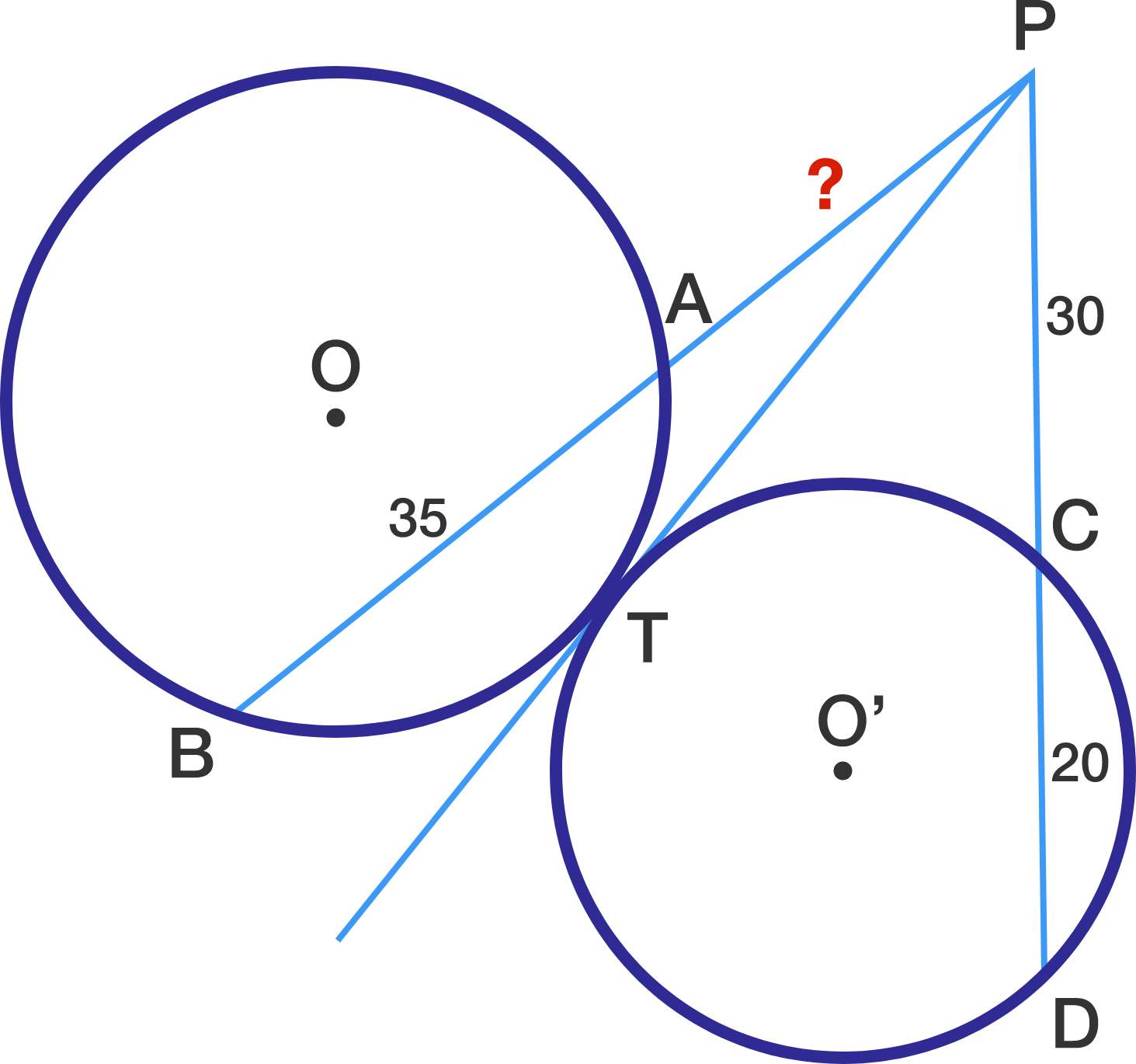# Tangents Secants and Circles...Oh My

Geometry Level 1In the above diagram, line segment $\overline{PT}$ is tangent to both circle $O$ and circle $O'.$ Given the following three lengths: $\lvert\overline{AB}\rvert = 35, \lvert\overline{PC}\rvert = 30, \lvert\overline{CD}\rvert = 20,$ what is $\lvert\overline{PA}\rvert?$

×